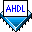## Using an If Then Statement vs. a Case Statement

 See AlsoIf Then and Case Statements are similar. In some cases, you can use either statement to achieve the same results. The following example shows the same operation expressed in both If Then and Case Statement formats:

 If Then Statement: Case Statement: ``` IF a[] == 0 THEN y = c & d; ELSIF a[] == 1 THEN y = e & f; ELSIF a[] == 2 THEN y = g & h; ELSIF a[] == 3 THEN y = i; ELSE y = GND; END IF; ``` ```CASE a[] IS WHEN 0 => y = c & d; WHEN 1 => y = e & f; WHEN 2 => y = g & h; WHEN 3 => y = i; WHEN OTHERS => y = GND; END CASE; ```

Important differences exist between If Then and Case Statements:

• Any kind of Boolean expression can be used in an If Then Statement. Each expression following an `IF` or `ELSIF` clause may be unrelated to the other expressions in the statement. In a Case Statement, however, a single Boolean expression is compared to a constant in each `WHEN` clause.

• Using the `ELSIF` clause can result in logic that is too complex for the Compiler, because each successive `ELSIF` clause must still test that the preceding `IF/ELSIF` clauses are false. The following example shows how the Compiler interprets an If Then Statement. If `a` and `b` are complex expressions, the inversion of each expression is likely to be even more complex.

 If Then Statement: Compiler Interpretation: ```IF a THEN c = d; ELSIF b THEN c = e; ELSE c = f; END IF; ``` ```IF a THEN c = d; END IF; IF !a & b THEN c = e; END IF; IF !a & !b THEN c = f; END IF; ```